Next: Cylindrical Cavities Up: Resonant Cavities and Waveguides Previous: Quality Factor of a

# Axially Symmetric Cavities

The rectangular cavity that we have just discussed has many features in common with axially symmetric cavities of arbitrary cross-section. In every axially symmetric cavity, the allowed values of the wave vector,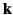, and thus the allowed frequencies, are determined by the cavity geometry. We have seen that for each set of mode numbers,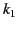,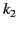,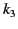, in a rectangular cavity, there are, in general, two linearly independent modes: that is, the polarization remains arbitrary. We can take advantage of this fact to classify modes into two types, according to the orientation of the field vectors. Let us choose one type of mode such that the electric field vector lies in the cross-sectional plane, and the other such that the magnetic field vector lies in this plane. This classification into transverse electric (TE) and transverse magnetic (TM) modes turns out to be possible for all axially symmetric cavities, although the rectangular cavity is unique in having one mode of each kind corresponding to each allowed frequency.

Suppose that the direction of symmetry is along the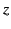-axis, and that the length of the cavity in this direction is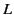. The boundary conditions at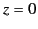and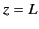demand that thedependence of wave quantities be either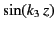or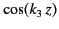, where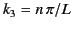. In other words, all wave quantities satisfy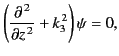(1327)

as well as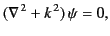(1328)

where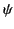stands for any component of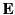or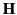. The field equations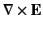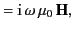(1329)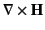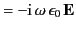(1330)

must also be satisfied.

Let us write each vector and each operator in the above equations as the sum of a transverse part, designated by the subscript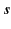, and a component along. We find that for the transverse fields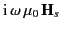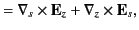(1331)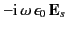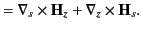(1332)

When one of Equations (1333)-(1334) is used to substitute for the transverse field on the right-hand side of the other, and use is made of Equation (1329), we obtain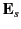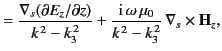(1333)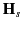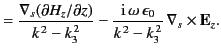(1334)

Thus, all transverse fields can be expressed in terms of thecomponents of the fields, each of which satisfies the differential equation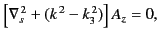(1335)

where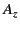stands for either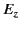or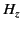, and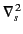is the two-dimensional Laplacian operator in the transverse plane.

The conditions onandat the boundary (in the transverse plane) are quite different:must vanish on the boundary, whereas the normal derivative ofmust vanish to ensure thatin Equation (1336) satisfies the appropriate boundary condition. If the cross-section is a rectangle then these two conditions lead to the same eigenvalues of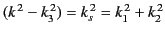, as we have seen. Otherwise, they correspond to two different sets of eigenvalues, one for whichis permitted but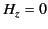, and the other where the opposite is true. In every case, it is possible to classify the modes as transverse magnetic or transverse electric. Thus, the field componentsandplay the role of independent potentials, from which the other field components of the TE and TM modes, respectively, can be derived using Equations (1335)-(1336).

The mode frequencies are determined by the eigenvalues of Equations (1329) and (1337). If we denote the functional dependence oforon the plane cross-section coordinates bythen we can write Equation (1337) as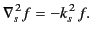(1336)

Let us first show that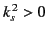, and, hence, that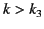. Now,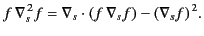(1337)

It follows that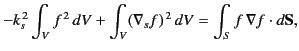(1338)

where the integration is over the transverse cross-section,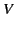. If either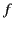or its normal derivative is to vanish on the conducting surface,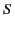, then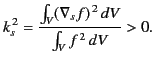(1339)

We have already seen that. The allowed values of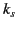depend both on the geometry of the cross-section, and the nature of the mode.

For TM modes,, and thedependence ofis given by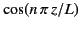. Equation (1338) must be solved subject to the condition thatvanish on the boundaries of the plane cross-section, thus completing the determination ofand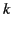. The transverse fields are then given by special cases of Equations (1335)-(1336):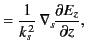(1340)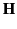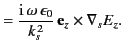(1341)

For TE modes, in which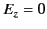, the condition thatvanish at the ends of the cylinder demands a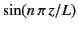dependence on, and awhich is such that the normal derivative ofis zero at the walls. Equations (1335)-(1336), for the transverse fields, then become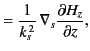(1342)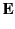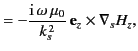(1343)

and the mode determination is complete.Next: Cylindrical Cavities Up: Resonant Cavities and Waveguides Previous: Quality Factor of a
Richard Fitzpatrick 2014-06-27